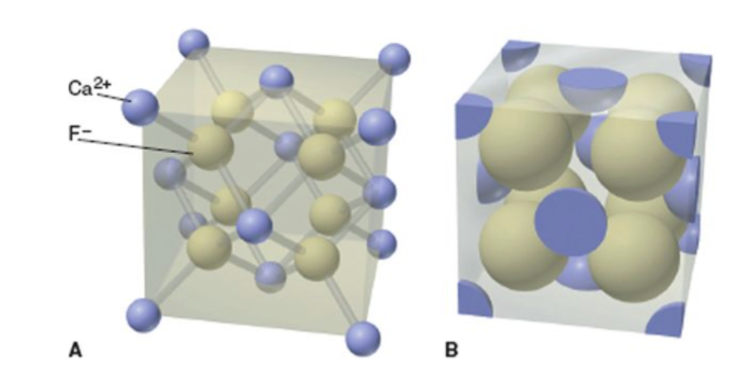# Problem: This picture shows the cubic unit cell of an ionic compound comprised of Ba2+, O- and Ti4+. Both pictures show the same cubic unit cell. What is the empirical formula based on the cubic unit cell. This picture shows the cubic unit cell of CaF2. How many Ca2+ ions are present in this cubic unit cell?How many F- ions are in this cubic unit cell?

###### FREE Expert Solution

In a unit cell: atoms have different contribution depending on their location

contribution
corner atoms                                 1/8
atoms in center/fully inside          1
atoms in face of the cube            1/2

96% (196 ratings)###### Problem Details

This picture shows the cubic unit cell of an ionic compound comprised of Ba2+, O- and Ti4+. Both pictures show the same cubic unit cell. What is the empirical formula based on the cubic unit cell.This picture shows the cubic unit cell of CaF2. How many Ca2+ ions are present in this cubic unit cell?

How many F- ions are in this cubic unit cell?

Frequently Asked Questions

What scientific concept do you need to know in order to solve this problem?

Our tutors have indicated that to solve this problem you will need to apply the Unit Cell concept. You can view video lessons to learn Unit Cell. Or if you need more Unit Cell practice, you can also practice Unit Cell practice problems.